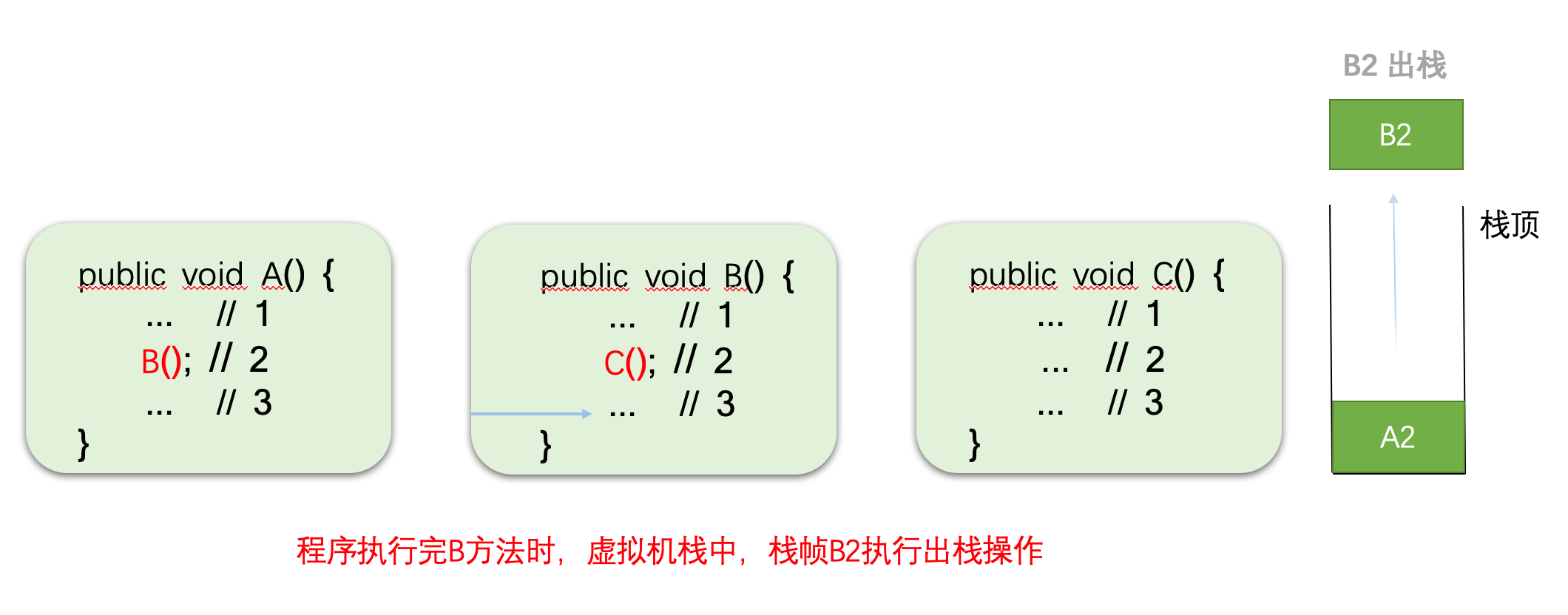Java堆栈

×

# Java堆栈

## 特点

• 只能从栈顶添加元素或者删除元素

• 后进先出的数据结构，Last In First Out(LIFO)

1. 入栈操作示意图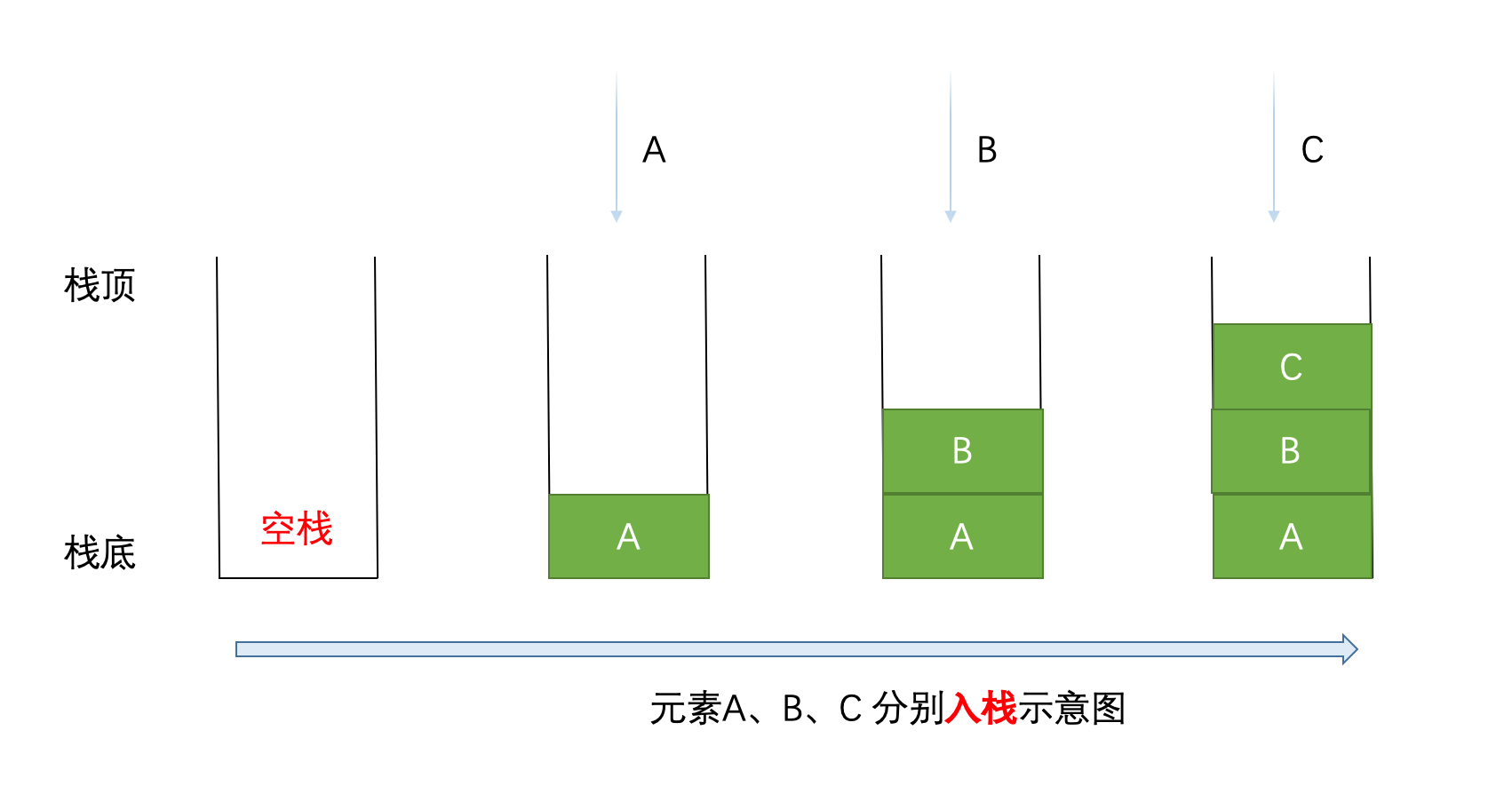2. 出栈操作示意图(后进的元素先出)• 向栈中添加一个元素(入栈)

``void push(E e)``

• 从栈中删除一个元素(出栈)

``E pop()``

• 查看栈顶元素

``E peek()``

• 查看栈中元素个数

``int getSize()``

• 判断栈是否为空

``boolean isEmpty()``

1. 接口定义：Stack<E>

``````public interface Stack<E> {

/**
* 向栈中添加元素
*
* @param e
*/
void push(E e);

/**
* 从栈中删除元素
*/
void pop();

/**
* 获取栈顶元素
*
* @return
*/
E peek();

/**
* 获取栈中元素个数
*
* @return
*/
int getSize();

/**
* 判断栈中是否为空
*
* @return
*/
boolean isEmpty();

}``````

``````public class LinkedListStack<E> implements Stack<E> {
/**
* 存放栈元素
*/

/**
* 构造栈结构
*/
}

@Override
public void push(E e) {
}

@Override
public void pop() {
list.removeLast();
}

@Override
public E peek() {
return list.getLast();
}

@Override
public int getSize() {
return list.size();
}

@Override
public boolean isEmpty() {
return list.isEmpty();
}

@Override
public String toString() {
"list=" + list +
'}';
}
}``````

``````@Test
// 栈
// 准备入栈元素
List<String> prepareElements = Arrays.asList("A", "B", "C", "D", "E");
// 入栈
prepareElements.forEach(x -> {
stack.push(x);
System.out.println("入栈操作：" + stack);
});
// 出栈
stack.pop();
System.out.println("出栈操作：" + stack);
// 获取栈顶元素
String peekElement = stack.peek();
System.out.println("栈顶元素：" + peekElement);
// 获取栈中元素的个数
int stackSize = stack.getSize();
System.out.println("栈中元素个数：" + stackSize);
}``````

4. 运行结果

``````入栈操作：LinkedListStack{list=[A]}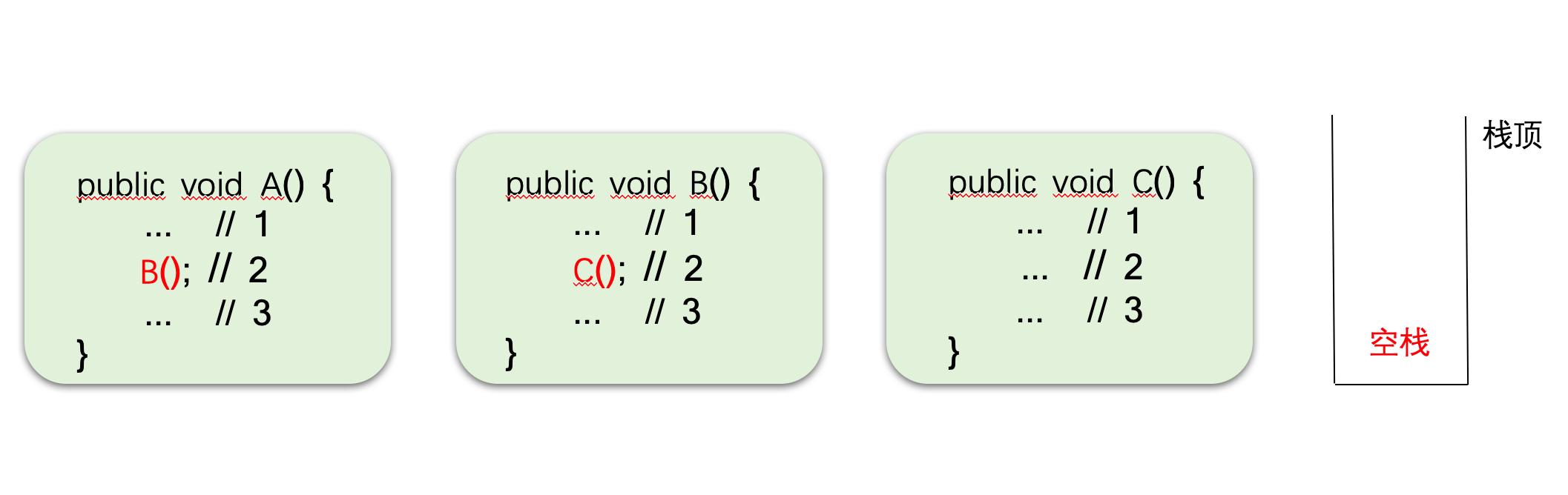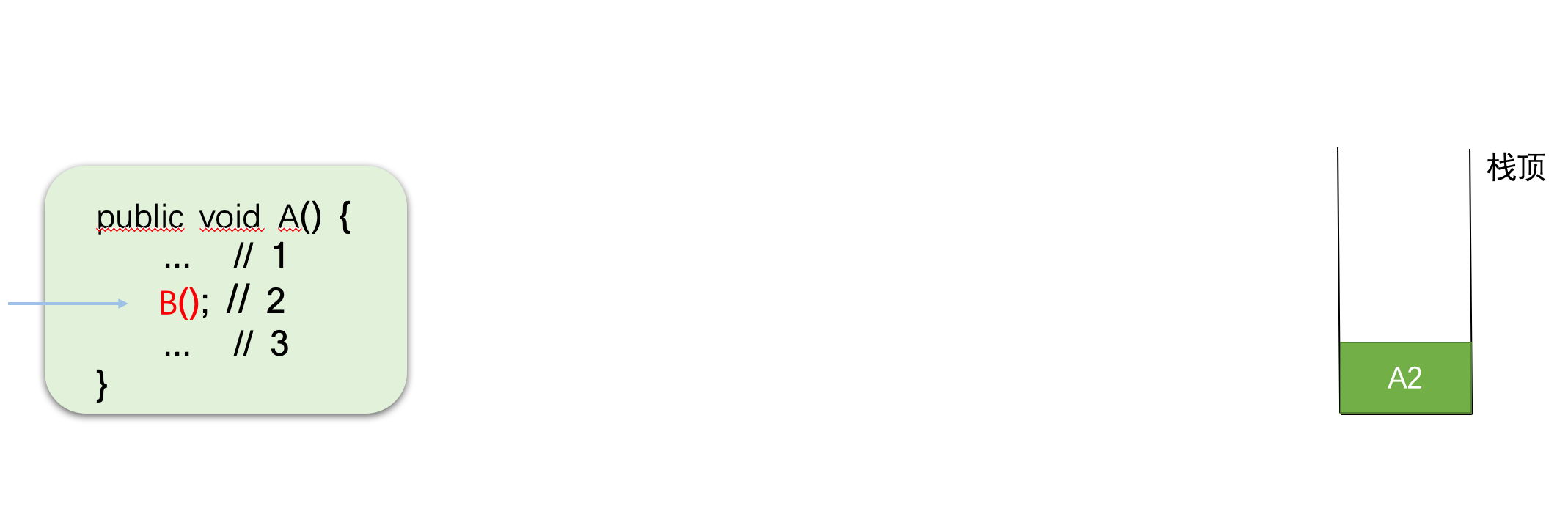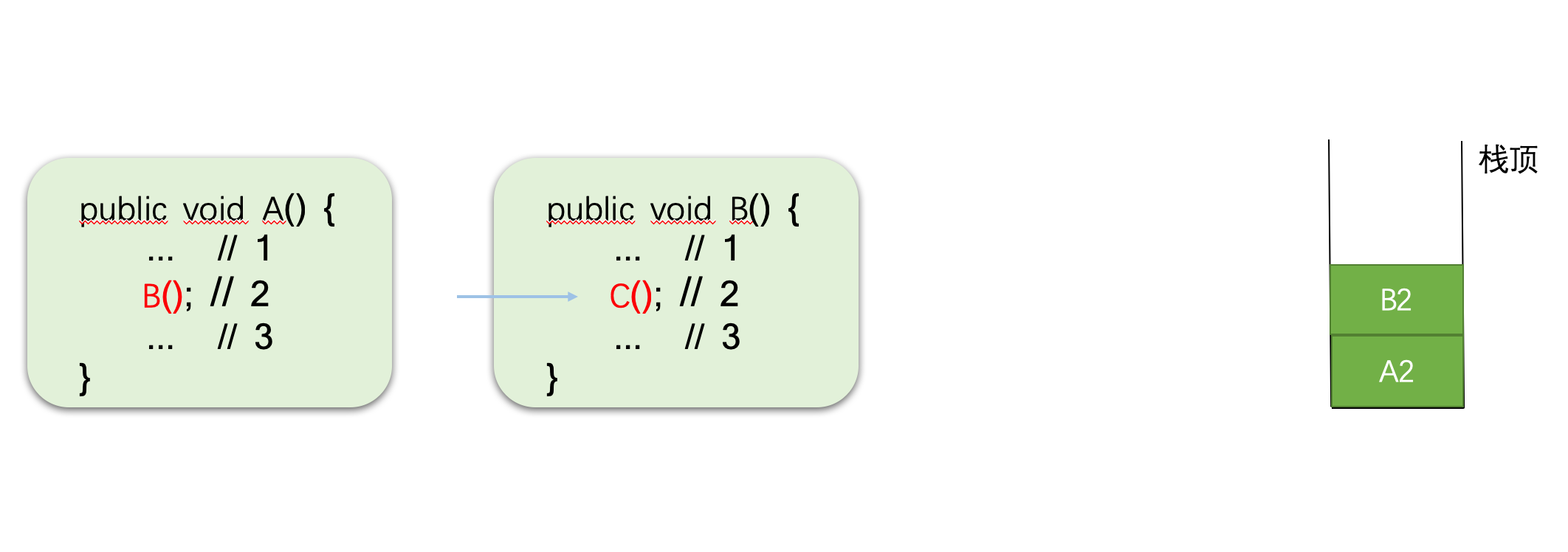当程序开始执行到C()方法时，直到执行完C()方法时，这时候，程序该如何执行呢?